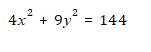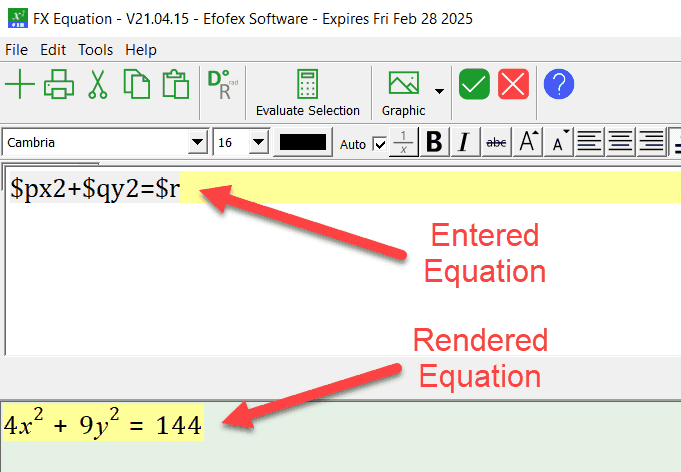﻿ Parameters

# Parameters

Top  Previous  Next
 Efofex Parameters provide you with a way of creating dynamic, mathematical materials and documents.   Parameters always begin with a \$ sign followed by a letter and then letters and numbers.. When you use them in an equation, they are automatically replaced by a number or string when the equation is rendered.   For example, you might type   \$px2+\$qy2=\$r   If you have previously defined the parameters \$p=4, \$q=9 and \$r=144, this might be rendered asIn FX Equation, this might look like thisFX Equation has replaced the three parameters with the three numbers. So far, so good, but this is not very "dynamic". Things begin to get dynamic when we start using the different types of parameters.